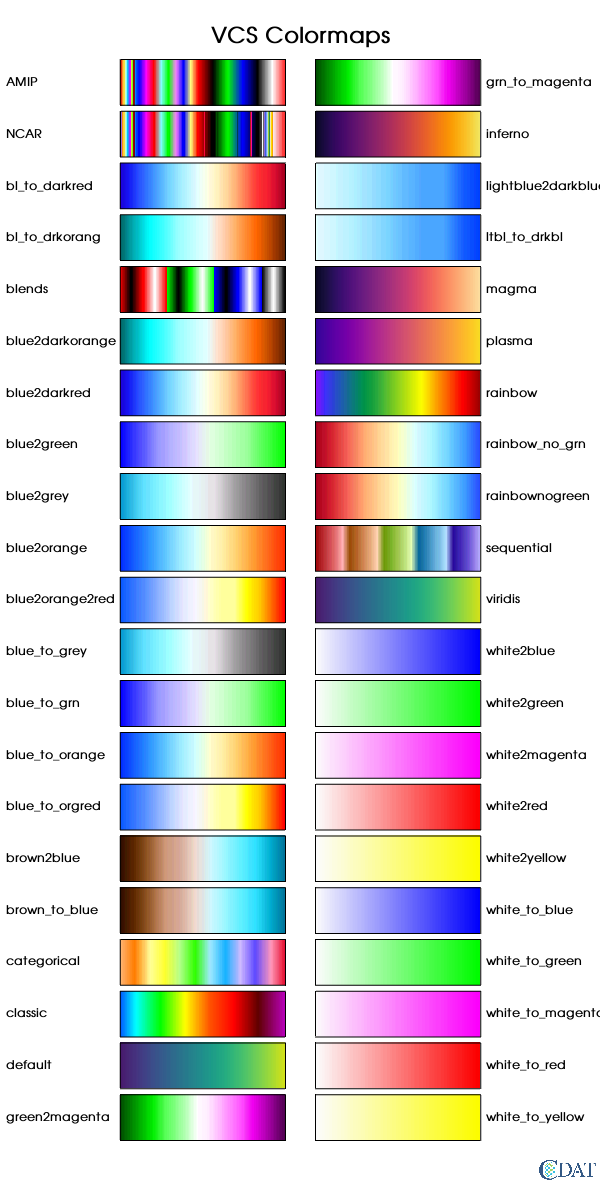# Color maps of UV-CDAT¶

Grep all available color maps and visualize them using EzTemplate

The CDAT software was developed by LLNL. This tutorial was written by Charles Doutriaux and Jiwoo Lee (2017). This work was performed under the auspices of the U.S. Department of Energy by Lawrence Livermore National Laboratory under Contract DE-AC52-07NA27344.

# Import modules¶

In :
import vcs
import MV2
import numpy as np

/Users/muryanto1/tempdir/vcs/miniconda3/envs/vcs_nightly/lib/python3.7/site-packages/unidata/__init__.py:2: UserWarning: unidata package is deprecated please use genutil.udunits instead of unidata.udunits


# Prepare a dummy array¶

• We need a 2-D dummy array that will contain monotonically increasing numbers each of two rows, to be used for the visualization
In :
a=[]
num_levels = 224

for i in range(0,num_levels):
a.append(i/float(num_levels))

for i in range(0,num_levels):
a.append(i/float(num_levels))

a = MV2.array(a)
a = MV2.reshape(a, (-1,num_levels))


# Colormap visualizing function¶

• Below function will visualize given colormaps to provide a selection guidance.
In :
import math

T = vcs.createtemplate()
T.blank(['title','mean','min','max','dataname','crdate','crtime',
'units','zvalue','tvalue','xunits','yunits','xname','yname', 'legend'])

cmlst_num = len(cmlst)
nrows = int(math.ceil(cmlst_num/2.))

M = EzTemplate.Multi(template=T, rows=nrows, columns=2)
M.margins.top = 0.05
M.margins.bottom = 0.05
M.margins.left = 0.2
M.margins.right = 0.2
M.spacing.vertical= 0.005

for i in range(0, cmlst_num):
box = v.createboxfill()
box.color_1 = 16
box.color_2 = 240
box.xticlabels('','') # Hide x-axis tick labels
box.yticlabels('','') # Hide y-axis tick labels

if i >= nrows: #right column
jj=1
ii=i-nrows
x = 0.81 # text starting x position
else: # left column
jj=0
ii=i
x = 0.01 # text starting x position

t = M.get(row=ii, column=jj, legend='none')
t.blank(['legend'])

v.plot(a, t, box, bg=1)

subplot_title = vcs.createtext()
subplot_title.string = box.colormap
subplot_title.x = x
subplot_title.y = (t.box1.y1 + t.box1.y2) / 2.
subplot_title.height = 8
subplot_title.halign = 'left'
subplot_title.valign = 'half'
subplot_title.color = 'black'
v.plot(subplot_title, t)
v.removeobject(box)
v.removeobject(subplot_title)

#print box.colormap  ## List available color maps
return(v)


# Open a VCS canvas¶

• Let's open a VCS canvas to play with!
In :
v = vcs.init(geometry={"width":600,"height":1200}, bg=1)
vcs.utils.defaultColorsRange = range(16,240)


# VCS colormaps¶

• VCS is default module that is being used for the UV-CDAT. You can use VCS color maps which is loaded at the beginning.
In :
cmlst = v.listelements('colormap')

plot_title = v.createtext()
plot_title.string = 'VCS Colormaps'
plot_title.height = 15
plot_title.halign = 'center'
plot_title.valign = 'bottom'
plot_title.x = 0.5
plot_title.y = 0.96
v.plot(plot_title)Out:
<vcs.displayplot.Dp at 0x11c256bb0>

# Matplotlib colormaps in VCS¶

• You can load and visualize Matplotlib colormaps in the VCS canvas.
• Use vcs.colors.matplotlib2vcs to convert
• For now if same name is already being used in VCS, new name with tail _000 is assigned, as showing below as "warning messages".
• You can also use vcs.utils.loadmatplotlibcolormaps() to bring all Matplotlib colormaps to VCS
In :
v.clear()

import matplotlib.pyplot as plt

# Get list of all available colormaps from matplotlib (except '_r' which is reversed one)
cmlst = [x for x in plt.colormaps() if not '_r' in x]
cmlst = sorted(cmlst, key=lambda s: s.lower()) # Sort as case-insensitive alphabet order


/Users/muryanto1/tempdir/vcs/miniconda3/envs/vcs_nightly/lib/python3.7/site-packages/vcs-v8.2_27_g1c84fa66-py2.7.egg/vcs/colors.py:43: UserWarning: inferno colormap name was already existing, your colormap name will be: inferno_mpl_000